# GED Math : Statistics

## Example Questions

← Previous 1 3 4 5 6 7 8 9 26 27

### Example Question #1 : Probability

When rolling a-sided die, what is the probability of rollingor greater?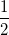Explanation:

When rolling a die, the following outcomes are possible: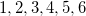Of theoutcomes,outcomes areor greater. Therefore,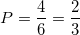### Example Question #1 : Probability

The two red queens are removed from a standard deck of 52 cards. What is the probability that a randomly dealt card from this altered deck will be red?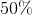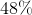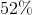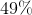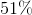Explanation:

After the removal of two red cards from a deck of 52, there are now fifty cards, of which twenty-four are red. The probability of a random card being red is therefore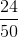, which as a percent is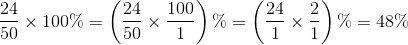### Example Question #1 : Calculations

What is the probability of drawing a red jack from a deck of standard playing cards?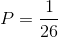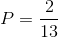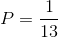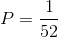Explanation:

A standard deck of play cards hascards. There arejacks,of which are red. Therefore, the probability is: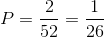### Example Question #1 : Calculations

Which of the following elements can be added to the data set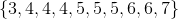so that its mode(s) remain unchanged?

I:II:III:I and III only

I, II, and III

II and III only

I and II only

I and III only

Explanation:

The mode of the data set is the most frequently occurring element. In the set given, 4 occurs three times, 5 occurs three times, 6 occurs two times, and 3 and 7 occur one time each. Therefore, the set has two modes, 4 and 5, and we want to preserve this condition.

If 3 is added to this set, it becomes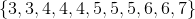and 4 and 5 are still tied for the most frequently occurring element. The same happens if  7 is added to yield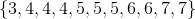.

If 5 is added to this set, it becomes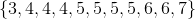and 5 appears more frequently than 4 or any other element. This changes the data set to one with only one mode, 5.

The correct response is therefore "I and III only".

### Example Question #5 : Calculations

Given the data set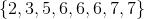, which of the following quantities are equal to each other/one another?

I: The mean

II: The median

III: The mode

I and II only

II and III only

I, II, and III

I and III only

II and III only

Explanation:

Since this data set is arranged in ascending order and has an even number of elements, the median of the data set is the arithmetic mean of its middle two elements. Both elements are 6, so this is the median.

6 is the mode, since it occurs most frequently.

The mean is the sum of the elements divided by the number of elements, which is 8: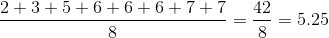The median and the mode are equal to each other, but not to the mean, so the correct answer is "II and III only".

### Example Question #1 : Probability

A red die is altered so that it comes up a "6" with probability. The other five numbers are equally likely outcomes to one another. A blue die is altered similarly. If these dice are rolled, what is the probability that the outcome will be a total of "11"?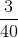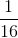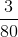Explanation:

For a roll of "11" to occur with two dice, one die must show a "5" and the other must show a "6". For each die, since a "6" will come up with probability, for the other five rolls to be equally likely, each, including "5", must come up with probability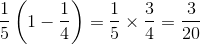.

The probability of rolling a red "5" and a blue "6" will be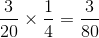which is also the probability of rolling a blue "5" and a red "6". Add: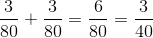### Example Question #1 : Calculations

Roll a fair dice.  Then flip a coin.  What's the probability of rolling a 3 or greater, and then flipping a heads?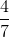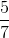Explanation:

There are 4 possibilities that a 3 or higher can be rolled on a die.

The probability of rolling a 3 or higher on a fair die is: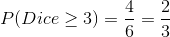Rolling a heads on a coin has a probability of one half.  Therefore,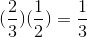### Example Question #2 : Probability

Seven boys and three girls are finalists in a drawing. The ten names are placed into a hat, and two are drawn at random. What is the probability that the names of one boy and one girl will be drawn?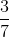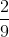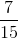Explanation:

Since order is irrelevant here, we are dealing with combinations.

There are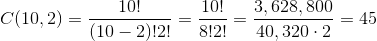ways to choose two out of ten students.

There are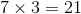ways to choose one boy and one girl.

The probability that one boy and one girl will be chosen is therefore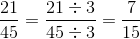.

### Example Question #1 : Calculations

Six boys and four girls are finalists in a drawing. The ten names are placed into a hat, and two are drawn at random. What is the probability that both names drawn will be girls?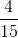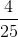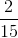Explanation:

Since order is irrelevant here, we are dealing with combinations.

There areways to choose two out of ten students.

There are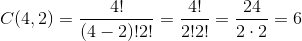ways to choose two out of four girls.

The probability that two girls will be chosen is therefore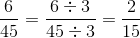.

### Example Question #1 : Probability

A penny is altered so that the odds are 5 to 4 against it coming up tails when tossed; a nickel is altered so that the odds are 4 to 3 against it coming up tails when tossed. If both coins are tossed, what are the odds of there being two heads or two tails?

11 to 10 against

32 to 31 in favor

32 to 31 against

11 to 10 in favor

32 to 31 in favor

Explanation:

5 to 4 odds in favor of heads is equal to a probability of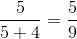, which is the probability that the penny will come up heads. The probability that the penny will come up tails is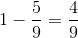.

Similarly, 4 to 3 odds in favor of heads is equal to a probability of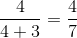, which is the probability that the nickel will come up heads. The probablity that the nickel will come up tails is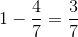.

The outcomes of the tosses of the penny and the nickel are independent, so the probabilities can be multiplied.

The probability of the penny and the nickel both coming up heads is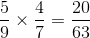.

The probability of the penny and the nickel both coming up tails is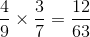.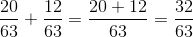Since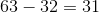, this translates to 32 to 31 odds in favor of this event.